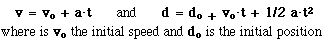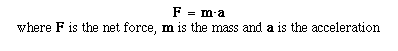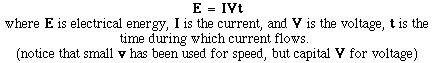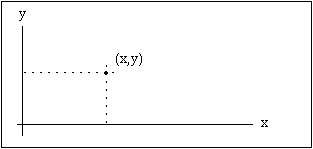# Hands-On-PhysicsMECHANICS

## - Mathematical Models -

### Equations

One of the main strengths of physics is its use of mathematical modelling.

The mathematical model for motion, begins with the definition of average speed and the definition of acceleration. Notice that the symbol v is usually used for speed. This tradition comes from the fact that speed is the size of the velocity vector.Rearrangements and combinations of these equations yield a set of relationships which describe motion whenever the acceleration is constant. Two of these relationships called the equations of motion allow the calculation of speed and position at any time if the initial position and speed are known.Whenever the speed change is steady, the acceleration is constant. There are many physical situations when acceleration is constant; an object falling in gravity, or pushed by a constant force. A constant force produces a constant acceleration (steady speed change). This relationship between force and motion has been formalized in Newton's Second Law.Newton's law in this form is used to define force, but the law is perhaps more intuitive when written it terms of the acceleration.When an unbalanced force acts on an object, the force does work and the object changes its speed. The work done by the net force depends simply on the force and the distance moved.The work done by the net force changes the kinetic energy of an object.Energy is often stored and moved around electrically. When energy carried by electricity, that energy is not measured directly, but may be determined from measurements of current, voltage, and time.### Graphs

Visualization is often helpful for the study of changing speed. Two dimensional graphs are used for displaying sets of number pairs which are related. Usually each of the ordered pairs (x,y) has one number which is predetermined by the experimenter, and one which is measured. The predetermined number, called the independent variable, is given a horizontal (x) position and its related measured number, called the dependent variable, is given a vertical (y) position.Figure P1
A Point in XY Space

Graphs of speed vs. time supplement and clarify the mathematical model of motion. For these graphs, most everybody is in the habit of using the horizontal axis for time. Mathematicians and scientists are in the habit of using the horizontal axis for the independent variable. These customs are in conflict when time is allowed to be dependent variable. We choose to plot time on the horizontal axis in keeping with ordinary habit, partly because this conveniently makes the slope of a time graph equal a rate of change. Time graphs are continuous functions which show how change plays out as time flows forward. The vertical location of a point on a time graph tells the size at that time, and the slope of a time graph tells the rate of change.Figure P2
Slope

On a graph of s vs time, the slope tells the rate at which s changes with time. If s represents position, then the slope is the speed.

Previous Page || Up a Level || Index